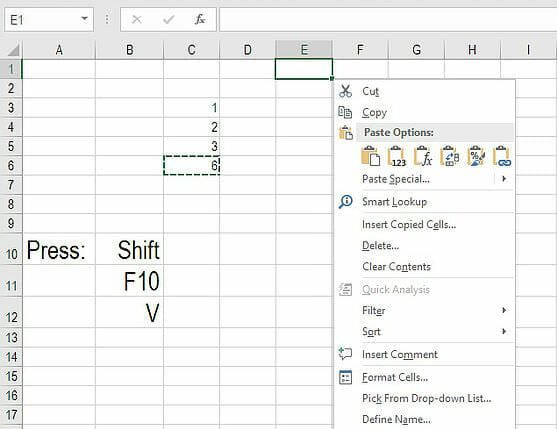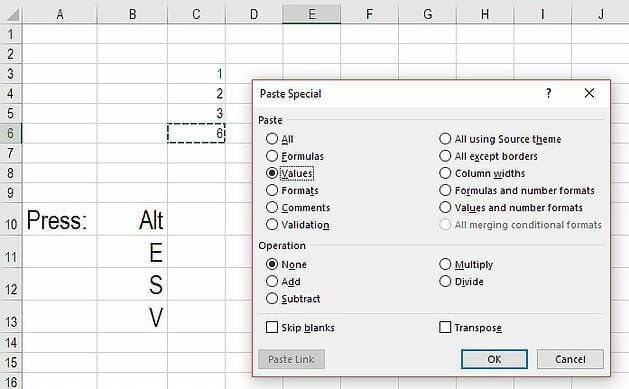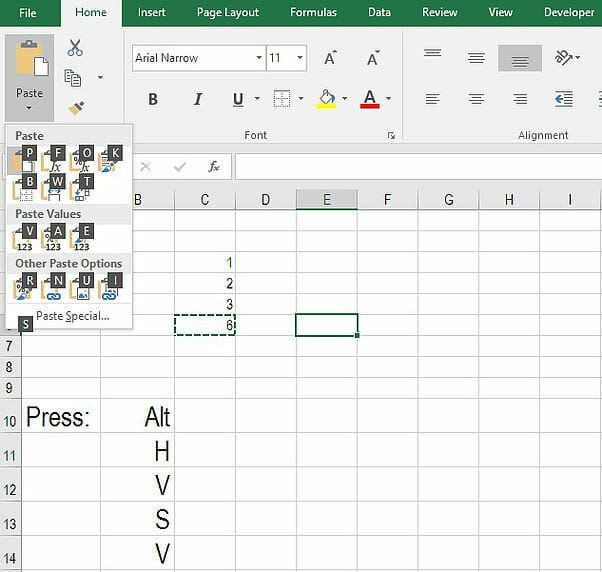# Excel Convert Formula to Value

Step-by-step guide to using shortcuts to convert formulas to values in Excel

## Convert formulas to values in Excel

There are three shortcuts you can use to convert formulas to values in Excel. This article on “Excel Convert Formula To Value” gives a step by step breakdown of each.

The first option, using Shift + F10 requires the fewest keystrokes; the second, Alt + E + S is slightly longer; and the third, using the ribbon shortcuts via Alt + H requires the most keystrokes. Below is a complete guide of how to use each approach to make formulas into values.

### Method 1: Shift, F10, V

1. Highlight the cells you want to convert
2. Press Ctrl + C
3. Press Shift + F10 and VLearn more shortcuts in our free Excel crash course.

### Method 2: Alt, E, S, V

1. Highlight the cells you want to convert
2. Press Ctrl + C
3. Press Alt + E + S + V### Method 3: Alt, H, V…

1. Highlight the cells you want to convert
2. Press Ctrl + C
3. Press Alt + H + V + S + VPaste Special Values

All three methods above leverage the Paste Special function in Excel. This function allows Excel users to paste the information in a variety of ways, including formatting, values, formulas, multiplication, division, and more. We encourage you to explore the various uses for Paste Special, in addition to converting a formula to value.

Launch Free Excel Course

### Applications of Excel Convert Formula To Value in financial modeling

Converting formulas to values in Excel is very handy in financial modeling. The most common use is performing side calculations to arrive at a value that’s used as an assumption. For example, imagine you have a list of assumptions about the number of customers per year in a model. You may want to quickly calculate how many customers you expect in 2019 in a separate area of the Excel model, and then copy that formula and paste it as a value in the model.

Launch Free Excel Course

### More Excel shortcuts

Thanks for reading our guide on “Excel Convert Formula To Value”. At CFI, we’ve taken great care to compile all the most important Excel shortcuts for aspiring analysts to advance their careers. To that end, we’ve listed below our most useful guides, templates, and resources on a wide range of Excel topics.

• All Excel shortcuts
• List of Excel formulas
• Financial modeling guide
• How to become a financial analyst

### Free Excel Tutorial

To master the art of Excel, check out CFI’s FREE Excel Crash Course, which teaches you how to become an Excel power user.  Learn the most important formulas, functions, and shortcuts to become confident in your financial analysis.

Launch CFI’s Free Excel Course now to take your career to the next level and move up the ladder!